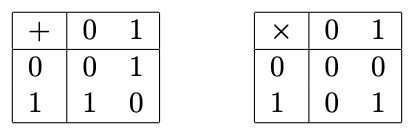시간 제한 메모리 제한 제출 정답 맞은 사람 정답 비율
3 초 512 MB 12 9 8 80.000%

## 문제

A finite field F2 consists of two elements: 0 and 1. Addition and multiplication on F2 are those on integers modulo two, as defined below.A set of vectors v1, . . . , vk over F2 with the same dimension is said to be linearly independent when, for c1, . . . , ckF2, c1v1 + · · · + ckvk = 0 is equivalent to c1 = · · · = ck = 0, where 0 is the zero vector, the vector with all its elements being zero.

The rank of a matrix is the maximum cardinality of its linearly independent sets of column vectors. For example, the rank of the matrix $\begin{bmatrix} 0 & 0 & 1 \\ 1 & 0 & 1 \end{bmatrix}$ is two; the column vectors $\begin{bmatrix} 0 \\ 1 \end{bmatrix}$ and $\begin{bmatrix} 1 \\ 1 \end{bmatrix}$ (the first and the third columns) are linearly independent while the set of all three column vectors is not linearly independent. Note that the rank is zero for the zero matrix.

Given the above definition of the rank of matrices, the following may be an intriguing question. How does a modification of an entry in a matrix change the rank of the matrix? To investigate this question, let us suppose that we are given a matrix A over F2. For any indices i and j, let A(ij) be a matrix equivalent to A except that the (i, j) entry is flipped.

$A_{kl}^{\left(ij\right)} = \begin{cases} A_{kl} + 1 & (k = i \text{ and } l = j) \\ A_{kl} & (\text{otherwise}) \end{cases}$

In this problem, we are interested in the rank of the matrix A(ij). Let us denote the rank of A by r, and that of A(ij) by r(ij). Your task is to determine, for all (i, j) entries, the relation of ranks before and after flipping the entry out of the following possibilities: (i) r(ij) < r, (ii) r(ij) = r, or (iii) r(ij) > r.

## 입력

The input consists of a single test case of the following format.

n m
A11 . . . A1m
.
.
.
An1 . . . Anm

n and m are the numbers of rows and columns in the matrix A, respectively (1 ≤ n ≤ 1000, 1 ≤ m ≤ 1000). In the next n lines, the entries of A are listed without spaces in between. Aij is the entry in the i-th row and j-th column, which is either 0 or 1.

## 출력

Output n lines, each consisting of m characters. The character in the i-th line at the j-th position must be either - (minus), 0 (zero), or + (plus). They correspond to the possibilities (i), (ii), and (iii) in the problem statement respectively.

## 예제 입력 1

2 3
001
101


## 예제 출력 1

-0-
-00


## 예제 입력 2

5 4
1111
1000
1000
1000
1000


## 예제 출력 2

0000
0+++
0+++
0+++
0+++


## 예제 입력 3

10 10
1000001001
0000010100
0000100010
0001000001
0010000010
0100000100
1000001000
0000010000
0000100000
0001000001


## 예제 출력 3

000-00000-
0-00000-00
00-00000-0
+00000+000
00-0000000
0-00000000
000-00000-
0-000-0-00
00-0-000-0
+00000+000


## 예제 입력 4

1 1
0


## 예제 출력 4

+